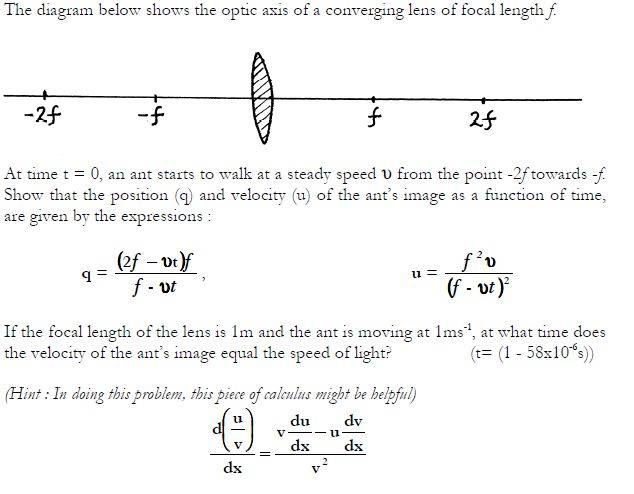# Position and Velocity as a function of time

## Homework Statement

At time t=0 an ant starts to walk from -2f to -f at a constant speed v. Show that the position (q) and velocity (u) of the ants image as a function of time is given by:

q=(2f-vt)f/(f-vt) AND u = f2v/(f-vt)2## Homework Equations

d(u/v)/dx = [V(du/dx)-U(dv/dx)]/v2

## The Attempt at a Solution

I am finding it hard to start the solution.

Last edited:

## Answers and Replies

haruspex
Science Advisor
Homework Helper
Gold Member
2020 Award
When the ant is at distance x from the lens, where is the image? You surely have a standard equation for that.

When the ant is at distance x from the lens, where is the image? You surely have a standard equation for that.

1/q=1/f+1/x
where x is the ants distance
q is the image distance and
f is the focal length

OR In newtonian terms:
X1 = S1 - f
X2 = S2 - f

Last edited:
haruspex
Science Advisor
Homework Helper
Gold Member
2020 Award
1/q=1/f+1/x
where x is the ants distance
q is the image distance and
f is the focal length
OK, so if you can express the ant's position as a function of time, you can use the above equation to get the position of the image as a function of time.